# Born-Oppenheimer approximation

In computational molecular physics and solid state physics the Born-Oppenheimer approximation is used to separate the quantum mechanical motion of the electrons from the motion of the nuclei. The method relies on the large mass ratio of electrons and nuclei. For instance the lightest nucleus, the hydrogen nucleus, is already 1836 times heavier than an electron. The method is named after Max Born and Robert Oppenheimer, who proposed it in 1927.

## Rationale

The computation of the energy and wave function of an average-size molecule is a formidable task that is alleviated by the Born-Oppenheimer (BO) approximation. For instance the benzene molecule consists of 12 nuclei and 42 electrons. The time-independent Schrödinger equation, which must be solved to obtain the energy and molecular wave function of this molecule, is a partial differential eigenvalue equation in 162 variables—the spatial coordinates of the electrons and the nuclei. The BO approximation makes it possible to compute the wave function in two less formidable, consecutive, steps. This approximation was proposed in the early days of quantum mechanics by Born and Oppenheimer (1927) and is indispensable in quantum chemistry and ubiquitous in large parts of computational physics.

In the first step of the BO approximation the electronic Schrödinger equation is solved, yielding a wave function depending on electrons only. For benzene this wave function depends on 126 electronic coordinates. During this solution the nuclei are fixed in a certain configuration, very often the equilibrium configuration. If the effects of the quantum mechanical nuclear motion are to be studied, for instance because a vibrational spectrum is required, this electronic computation must be repeated for many different nuclear configurations. The set of electronic energies thus computed becomes a function of the nuclear coordinates. In the second step of the BO approximation this function serves as a potential in a Schrödinger equation containing only the nuclei—for benzene an equation in 36 variables.

The success of the BO approximation is due to the high ratio between nuclear and electronic masses. The approximation is an important tool of quantum chemistry, without it only the lightest molecule, H2, could be handled; all computations of molecular wave functions for larger molecules make use of it. Even in the cases where the BO approximation breaks down, it is used as a point of departure for the computations.

## Short description

The Born-Oppenheimer (BO) approximation consists of two steps:

In the first step the nuclear kinetic energy Tnuc is omitted, that is, Tnuc is subtracted from the total molecular Hamiltonian. In the remaining electronic Hamiltonian Helec the nuclear positions still appear as fixed parameters in the attractions between nuclei and electrons. The electron-nucleus attractions are not removed; the electrons still feel the Coulomb potential of the nuclei clamped at certain positions in space. This first step of the BO approximation is therefore often referred to as the clamped nuclei approximation.

The electronic Schrödinger equation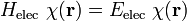$H_\mathrm{elec} \; \chi(\mathbf{r}) = E_\mathrm{elec} \; \chi(\mathbf{r})$

is solved (out of necessity approximately) with a fixed nuclear geometry as input. The quantity r stands for all electronic coordinates. Obviously, the electronic energy eigenvalue Eelec depends on the chosen positions R of the nuclei. Varying these positions R in small steps and repeatedly solving the electronic Schrödinger equation, one obtains Eelec as a function of R. This is the potential energy surface (PES): Eelec(R). Because this procedure of recomputing the electronic wave functions as a function of an infinitesimally changing nuclear geometry is reminiscent of the conditions for the adiabatic theorem, this manner of obtaining a PES is often referred to as the adiabatic approximation and the PES itself is called an adiabatic surface. The electronic energies Eelec(R) contain contributions of electron kinetic energies, interelectronic repulsions and electron-nuclear attractions. In a hand waving manner it is sometimes suggested that the nuclear potential is some averaged electron-nuclear attraction. This interpretation of the Born-Oppenheimer approximation is misleading.

In the second step of the BO approximation the nuclear kinetic energy Tnuc (containing partial derivatives with respect to the components of R) is reintroduced and added to the PES Eelec(R). Then the Schrödinger equation for the nuclear motion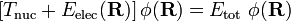$\left[ T_\mathrm{nuc} + E_\mathrm{elec}(\mathbf{R})\right] \phi(\mathbf{R}) = E_\mathrm{tot}\; \phi(\mathbf{R})$

is solved. This second step of the BO approximation involves separation of vibrational, translational, and rotational motions of the nuclei. This separation can be achieved by application of the Eckart conditions. The eigenvalue Etot is the total energy of the molecule, including contributions from electrons, nuclear vibrations, and overall rotation and translation of the molecule.

### Remark

Sometimes the BO approximation is presented in a simple, but erroneous, way. The Hamiltonian is incorrectly assumed to be separable in a nuclear and electronic part, which implies that the total wave function can be written as a product of a function depending on electrons only times a function depending on nuclei only:$\Psi_\mathrm{tot}(\mathbf{r,R}) = \psi_\mathrm{elec}(\mathbf{r}) \times \psi_\mathrm{nuc}(\mathbf{R})$.

This is incorrect, because, as was pointed out above, the Coulombic electron-nucleus attraction terms are not removed from the electronic Hamiltonian, so that$H_\mathrm{total} \ne H_\mathrm{elec} + H_\mathrm{nuc} \quad\hbox{and}\quad \Psi_\mathrm{tot}(\mathbf{r,R}) \ne \psi_\mathrm{elec}(\mathbf{r}) \times \psi_\mathrm{nuc}(\mathbf{R}).$

The essential feature of the factorization is that the wave function χk(r; R) introduced below depends on both the electron coordinates r and the nuclear coordinates R.

### Footnotes

1. This step is often justified by stating that "the heavy nuclei move more slowly than the light electrons." Classically this statement makes sense only if one assumes in addition that the momentum p of electrons and nuclei is of the same order of magnitude. In that case mnuc >> melec implies p2/(2mnuc) << p2/(2melec). Quantum mechanically it is not unreasonable to assume that the momenta of the electrons and nuclei in a molecule are comparable in magnitude—recall that the corresponding operators do not contain mass and think of the molecule as a box containing the electrons and nuclei and see particle in a box. Kinetic energy being p2/(2m), it follows that, indeed, the kinetic energy of the nuclei is much smaller than the kinetic energy of the electrons (the mass ratio being on the order of 104), so that neglect of nuclear kinetic energy is a reasonable first approximation.
2. It is assumed, in accordance with the adiabatic theorem, that the same electronic state (for instance the electronic ground state) is obtained upon small changes of the nuclear geometry. The method would give a discontinuity (jump) in the PES if electronic state-switching would occur.
3. This equation is time-independent and stationary wave functions for the nuclei are obtained, nevertheless it is traditional to use the word "motion" in this context, although classically motion implies time-dependence.
4. Wikipedia article retrieved 13 April 2009

## Derivation of the Born-Oppenheimer approximation

It will be discussed how the BO approximation may be derived and under which conditions it is applicable. At the same time we will show how the BO approximation may be improved by including vibronic coupling. To that end the second step of the BO approximation is generalized to a set of coupled eigenvalue equations depending on nuclear coordinates only. Off-diagonal elements in these equations are shown to be nuclear kinetic energy terms. It will be shown that the BO approximation can be trusted whenever the PESs, obtained from the solution of the electronic Schrödinger equation, are well separated:$E_0(\mathbf{R}) \ll E_1(\mathbf{R}) \ll E_2(\mathbf{R}), \cdots$ for all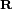$\mathbf{R}$.

The point of departure is the total (electrons and nuclei) exact non-relativistic, time-independent molecular Hamiltonian (from hereon the notation is shortened, nuc to n and elec to e):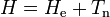$H= H_\mathrm{e} + T_\mathrm{n} \,$

with$H_\mathrm{e}= -\sum_{i}{\frac{1}{2}\nabla_i^2}- \sum_{i,A}{\frac{Z_A}{r_{iA}}} + \sum_{i>j}{\frac{1}{r_{ij}}}+ \sum_{A > B}{\frac{Z_A Z_B}{R_{AB}}} \quad\mathrm{and}\quad T_\mathrm{n}=-\sum_{A}{\frac{1}{2M_A}\nabla_A^2}.$

The position vectors r ≡ {ri} of the electrons and the position vectors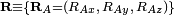$\mathbf{R}\equiv \{\mathbf{R}_A = (R_{Ax},\,R_{Ay},\,R_{Az})\}$ of the nuclei are with respect to a Cartesian inertial frame. Distances between particles are written as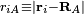$r_{iA} \equiv |\mathbf{r}_i - \mathbf{R}_A|$ (distance between electron i and nucleus A) and similar definitions hold for rij and RAB Let the molecule be in a homogeneous (no external force) and isotropic (no external torque) space and let the only interactions be the Coulomb interactions between the electrons and nuclei. The Hamiltonian is expressed in atomic units, so that the formula does not contain explicitly Planck's constant, the electric constant, the electronic charge, or the electronic mass. The only constants explicitly entering the formula are ZA and MA—the atomic numbers and masses of the nuclei.

It is useful to introduce the total nuclear momentum PA of nucleus A and to rewrite the nuclear kinetic energy operator as follows: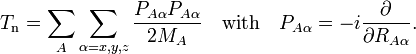$T_\mathrm{n} = \sum_{A} \sum_{\alpha=x,y,z} \frac{P_{A\alpha} P_{A\alpha}}{2M_A} \quad\mathrm{with}\quad P_{A\alpha} = -i {\partial \over \partial R_{A\alpha}}.$

Suppose we have K electronic eigenfunctions χk(r;R) of He, that is, we have solved$H_\mathrm{e}\;\chi_k (\mathbf{r};\mathbf{R}) = E_k(\mathbf{R})\;\chi_k (\mathbf{r};\mathbf{R}) \quad\mathrm{for}\quad k=1,\ldots, K.$

The electronic wave functions χk(r;R) will be taken to be real, which is possible when there are no magnetic or spin interactions. Strictly speaking, Ek(R) needs the label "e" as it is an electronic eigenvalue; for convenience the label is dropped since its dependence on R makes it clear that Ek(R) is the kth PES obtained from the solution of the electronic clamped nucleus equation.

The parametric dependence of the functions χk(r;R) on the nuclear coordinates is indicated by the symbol after the semicolon. This indicates that, although χk is a real-valued function of r, its functional form depends on R. For example, in the molecular-orbital-linear-combination-of-atomic-orbitals (LCAO-MO) approximation, χk is an MO given as a linear expansion of atomic orbitals (AOs). An AO depends visibly on the coordinates of an electron, but the nuclear coordinates are not explicit in the MO. However, upon change of geometry, i.e., change of R, the LCAO coefficients obtain different values and we see corresponding changes in the functional form of the MO χk. It is assumed that the parametric dependence is continuous and differentiable, so that it is meaningful to consider$P_{A\alpha}\chi_k (\mathbf{r};\mathbf{R}) = - i \frac{\partial\chi_k (\mathbf{r};\mathbf{R})}{\partial R_{A\alpha}} \quad \mathrm{for}\quad \alpha=x,y,z,$

which in general will not be zero. Note, parenthetically, that when the common error, pointed out above, is made, namely that χk does not depend on R, we find zero at this point, and the remaining derivation becomes much simpler (but wrong!).

The total wave function$\Psi(\mathbf{R},\mathbf{r})$ is expanded in terms of$\chi_k (\mathbf{r}; \mathbf{R})$: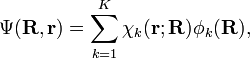$\Psi(\mathbf{R}, \mathbf{r}) = \sum_{k=1}^K \chi_k(\mathbf{r};\mathbf{R}) \phi_k(\mathbf{R}) ,$

with$\langle\,\chi_{k'}(\mathbf{r};\mathbf{R})\,|\, \chi_k(\mathbf{r};\mathbf{R})\rangle_{(\mathbf{r})} = \delta_{k' k}$

and where the subscript (r) indicates that the integration, implied by the bra-ket notation, is over electronic coordinates only. By definition, the matrix with general element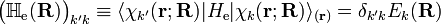$\big(\mathbb{H}_\mathrm{e}(\mathbf{R})\big)_{k'k} \equiv \langle \chi_{k'}(\mathbf{r};\mathbf{R}) | H_\mathrm{e} | \chi_k(\mathbf{r};\mathbf{R})\rangle_{(\mathbf{r})} = \delta_{k'k} E_k(\mathbf{R})$

is diagonal. After multiplication by the real function χk(r;R) from the left and integration over the electronic coordinates r, the total Schrödinger equation$H\;\Psi(\mathbf{R},\mathbf{r}) = E \; \Psi(\mathbf{R},\mathbf{r})$

is turned into a set of K coupled eigenvalue equations depending on nuclear coordinates only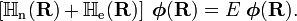$\left[ \mathbb{H}_\mathrm{n}(\mathbf{R}) + \mathbb{H}_\mathrm{e}(\mathbf{R}) \right] \; \boldsymbol{\phi}(\mathbf{R}) = E\; \boldsymbol{\phi}(\mathbf{R}).$

The column vector$\boldsymbol{\phi}(\mathbf{R})$ has elements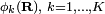$\phi_k(\mathbf{R}),\; k=1,\ldots,K$. The matrix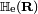$\mathbb{H}_\mathrm{e}(\mathbf{R})$ is diagonal and the nuclear Hamilton matrix is non-diagonal with the following off-diagonal (vibronic coupling) terms,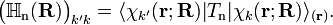$\big(\mathbb{H}_\mathrm{n}(\mathbf{R})\big)_{k'k} = \langle\chi_{k'}(\mathbf{r};\mathbf{R}) | T_\mathrm{n}|\chi_k(\mathbf{r};\mathbf{R})\rangle_{(\mathbf{r})}.$

The vibronic coupling in this approach is through nuclear kinetic energy terms. Solution of these coupled equations gives an approximation for energy and wave function that goes beyond the Born-Oppenheimer approximation. Unfortunately, the off-diagonal kinetic energy terms are usually difficult to handle. This is why often a diabatic transformation is applied, which retains part of the nuclear kinetic energy terms on the diagonal, removes the kinetic energy terms from the off-diagonal and creates coupling terms between the adiabatic PESs on the off-diagonal.

If we can neglect the off-diagonal elements the equations will uncouple and simplify drastically. In order to show when this neglect is justified, we suppress the coordinates in the notation and write, by applying the Leibniz rule for differentiation, the matrix elements of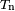$T_{\mathrm{n}}$ as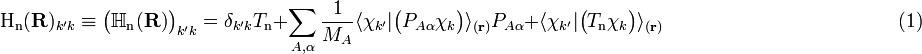$\mathrm{H_n}(\mathbf{R})_{k'k}\equiv \big(\mathbb{H}_\mathrm{n}(\mathbf{R})\big)_{k'k} = \delta_{k'k} T_{\textrm{n}} + \sum_{A,\alpha}\frac{1}{M_A} \langle\chi_{k'}|\big(P_{A\alpha}\chi_k\big)\rangle_{(\mathbf{r})} P_{A\alpha} + \langle\chi_{k'}|\big(T_\mathrm{n}\chi_k\big)\rangle_{(\mathbf{r})} \qquad\qquad\qquad\qquad\qquad\qquad(1)$

The diagonal k′ ≠ k matrix elements$\langle\chi_{k}|\big(P_{A\alpha}\chi_k\big)\rangle_{(\mathbf{r})}$ of the operator$P_{A\alpha}\,$ vanish, because this operator is Hermitian and purely imaginary. The off-diagonal matrix elements satisfy$\langle\chi_{k'}|\big(P_{A\alpha}\chi_k\big)\rangle_{(\mathbf{r})} = \frac{\langle\chi_{k'} |\big[P_{A\alpha}, H_\mathrm{e}\big] | \chi_k\rangle_{(\mathbf{r})}} {E_{k}(\mathbf{R})- E_{k'}(\mathbf{R})}.$

The matrix element in the numerator is$\langle\chi_{k'} |\big[P_{A\alpha}, H_\mathrm{e}\big] | \chi_k\rangle_{(\mathbf{r})} = iZ_A\sum_i \;\langle\chi_{k'}|\frac{(\mathbf{r}_{iA})_\alpha}{r_{iA}^3}|\chi_k\rangle_{(\mathbf{r})} \;\;\mathrm{with}\;\; \mathbf{r}_{iA} \equiv \mathbf{r}_i - \mathbf{R}_A .$

The matrix element of the one-electron operator appearing on the right hand side is finite. When the two surfaces come close,${E_{k}(\mathbf{R})\approx E_{k'}(\mathbf{R})},$ the nuclear momentum coupling term becomes large and is no longer negligible. This is the case where the BO approximation breaks down and a coupled set of nuclear motion equations must be considered, instead of the one equation appearing in the second step of the BO approximation.

Conversely, if all surfaces are well separated, all off-diagonal terms can be neglected and hence the whole matrix of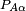$P_{A\alpha}$ is effectively zero. The third term on the right hand side of Eq. (1) (the Born-Oppenheimer diagonal correction) can approximately be written as the matrix of$P_{A\alpha}$ squared and, accordingly, is then negligible also. Only the first (diagonal) kinetic energy term in this equation survives in the case of well-separated surfaces and a diagonal, uncoupled, set of nuclear motion equations results,$\left[ T_\mathrm{n} +E_k(\mathbf{R})\right] \; \phi_k(\mathbf{R}) = E \phi_k(\mathbf{R}) \quad\mathrm{for}\quad k=1,\ldots, K,$

which are the normal second-step of the BO equations discussed above.

We reiterate that when two or more potential energy surfaces approach each other, or even cross, the Born-Oppenheimer approximation breaks down and one must fall back on the coupled equations. Usually one invokes then the diabatic approximation.

### Historical note

The Born-Oppenheimer approximation is named after M. Born and R. Oppenheimer who wrote a paper [Annalen der Physik, vol. 84, pp. 457-484 (1927)] entitled: Zur Quantentheorie der Molekeln (On the Quantum Theory of Molecules). This paper describes the separation of electronic motion, nuclear vibrations, and molecular rotation. A reader of this paper who expects to find clearly delineated the BO approximation—as it is explained above and in most modern textbooks—will be disappointed. The presentation of the BO approximation is well hidden in Taylor expansions (in terms of internal and external nuclear coordinates) of (i) electronic wave functions, (ii) potential energy surfaces and (iii) nuclear kinetic energy terms. Internal coordinates are the relative positions of the nuclei in the molecular equilibrium and their displacements (vibrations) from equilibrium. External coordinates are the position of the center of mass and the orientation of the molecule. The Taylor expansions complicate the theory tremendously and make the derivations very hard to follow. Moreover, knowing that the proper separation of vibrations and rotations was not achieved in this work, but only eight years later [by C. Eckart, Physical Review, vol. 46, pp. 383-387 (1935)] (see Eckart conditions), chemists and molecular physicists are not very much motivated to invest much effort into understanding the work by Born and Oppenheimer, however famous it may be. Although the article still collects many citations each year, it is safe to say that it is not read anymore, except maybe by historians of science.